## December 30, 2015

### How to sprintf a float with Arduino

Arduino has a small limitation in sprintf function that does not print correctly float numbers.
The following test sketch demonstrates the problem

```void setup()
{
Serial.begin(115200);
}

void loop()
{
float f = 123.12F;
Serial.print("Original value: ");
Serial.println(f);

char str;
sprintf(str, "String value: %f", f);
Serial.println(str);

Serial.println();
delay(2000);
}```

You can see from the screenshot below that the float is always formatted a question mark '?'.

### Using integer trick

There is a little trick to correctly print the float value using sprintf.

`sprintf(str, "String value: %d.%02d", (int)f, (int)(f*100)%100);`

This trick works well only with positive numbers and formats the float with two fixed decimals.

### Using dtostrf function

A better solution is to use the dtostrf function. It it is more flexible and works well also with negative numbers.
These two lines of code will write a string into the buffer with strcpy function and the append the float value using the dtostrf function.

```strcpy(str, "String value using dtostrf: ");
dtostrf(f, 2, 2, &str[strlen(str)]);```

#### 13 comments:

1.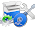Programming is very interesting and creative thing if you do it with love. Your blog code helps a lot to beginners to learn programming from basic to advance level. I really love this blog because I learn a lot from here and this process is still continuing.
Love from Pro Programmer

2.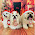This comment has been removed by the author.

3.This comment has been removed by the author.

4.The integer method is neat, but it has a little quirk: 12.4 is printed as "12.40" (it's OK) and 12.04 is printed as "12.4" (and it is confusing)

1.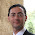You are right the example is not working perfectly.
This should work.
sprintf(str, "String value: %d.%02d", (int)f, (int)(f*100)%100);

I have corrected the example.
Thank you.

5.for negative floats just use an abs()-fuction:
sprintf(str, "String value: %d.%02d", (int)f, abs((int)(f*100)%100));

1.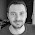with this method value from 0 and -0.9 are converted to positive.

6.Hello,

How is it possible to append in the same buffer more values

e.g

strcpy(str, "Sensor 1 value: "); //put a string into the str buffer
dtostrf(value, 2, 2, &str[strlen(str)]); //append the float value in the buffer
strcpy(str, "Sensor 2 value: "); //put a string into the str buffer
dtostrf(value1, 2, 2, &str[strlen(str)]); //append the float value in the buffer

without the second one overwriting the first?

Thank you!

7.strcpy(str, "Sensor 1 value: "); //put a string into the str buffer
dtostrf(value, 2, 2, &str[strlen(str)]); //append the float value in the buffer
strcat(str, " Sensor 2 value: "); //append a string to the end of str buffer
dtostrf(value1, 2, 2, &str[strlen(str)]); //append the float value in the buffer

do make sure str has enough space defined to hold all of appended strings otherwise you will have memory corruption leading to some very annoying things without warnings!
e.g. define str as: char str;
being "Sensor 1 value: " = 16
"-00.00" = 6
" Sensor 2 value: " = 17
"-00.00" = 6
---- +
total size = 45

1.46.
+1 for null character

8.thanks to unknown! exactly what i was looking for.

9.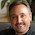Thanks YAAB, you solved my problem neatly. It is actually easy to make the first example work with negative values. The problem is that with a -ve the part of the result after the '.' gets its own '-', which we don't want. To solve this just "absolutize" the decimal part argument to sprintf...

sprintf(str, "String value: %d.%02d", (int)f, (int)(f < 0.0 ? -1 : 1) * (f*100)%100);

10.i tried this i cannot compile, below is my line.

sprintf(payload, " %d.%02d", (int)v, (int)(v < 0.0 ? -1 : 1) * (v*100)%100);

It says below, appreciate your help. thanks

exit status 1
invalid operands of types 'float' and 'int' to binary 'operator%'